## How should non-scale data be analyzed?

How should the non-scale data in the questionnaire be analyzed?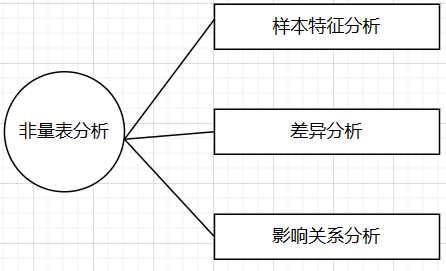1. Sample feature analysis
can use frequency analysis or visual graphics to describe non-scale questions. For example, multiple-choice questions can also be displayed using column charts. Through the results display, you can understand the basic situation of the sample, and finally make suggestions based on the analysis results, etc. .
2. Difference analysis
In addition, the difference relationship between samples can also be studied. This step can be combined with demographic variables, such as age, gender, and education. The difference method used in general non-scale analysis is chi-square test. Chi-square analysis is also called cross-tab analysis. It generally judges the difference by analyzing the relative selection frequency and proportion of different types of data. Single-choice questions and multiple-choice questions can also use chi-square analysis for comparative difference analysis. From the perspective of multiple-choice questions, the general chi-square analysis can be divided into two categories. One is the single-choice question chi-square analysis and the multiple-choice question chi-square analysis. Described below.
3. Impact relationship analysis

Non-scale data may also design impact relationship research, such as studying the influence of relevant factors on the sample group on the purchase of courses, you can consider using regression analysis, but if the dependent variable is a categorical (categorical) variable, you can use logit regression analysis. Generally, logit regression analysis can be divided into three types, binary logit regression analysis, multi-class logit regression analysis and ordered logit regression analysis.

## 1. Analysis of sample characteristics

Make a basic description of the non-scale data and get the corresponding conclusions. Take an example to explain the following. This case is to study the influence of various factors on an online English learning website on the willingness to purchase courses, and initially formulate research products, promotions, and channels. The impact of the six factors of promotion, price, personalized service and privacy protection on consumers' purchase intention. These include scale questions and demographic variables ( there are questionnaire descriptions and case data at the end of the article ). For example, if you want to study the distribution of the monthly income level of the respondents, the results are as follows: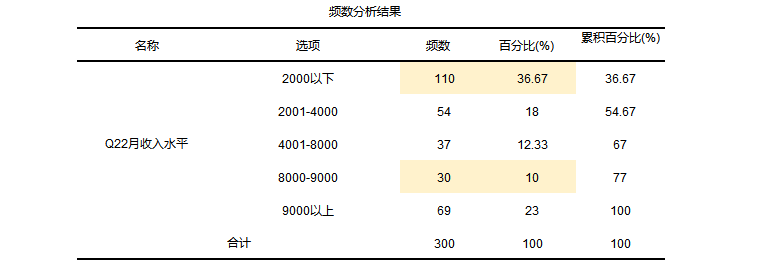From the results, we can get a total of 300 respondents, of which 110 have a monthly income of less than 2,000 yuan, accounting for 36.67% of the total. It can be seen that there is still a gap in income, which can be further analyzed in the follow-up analysis.

## 2. Difference analysis

For multiple-choice question types, chi-square analysis can be divided into two categories: one is single-choice chi-square test and the other is multiple-choice chi-square analysis. Generally, it is more complicated to perform chi-square analysis on multiple-choice questions.

1. Chi-square analysis of multiple choice questions

Chi-square analysis is to study the comparison of the differences between two categorical variables. It is to add statistical tests (chi-square value and p-value) on the basis of crossover, judge the p-value of the analysis results, and then explain whether the two categorical variables have Connections, such as whether there is a connection between gender and monthly income, etc. In the analysis, first judge the p value. If the p value is less than the significant level, it means that it is significant at the significant level. For example, if you want to study "whether there is a difference between gender and occupation".The result is as follows: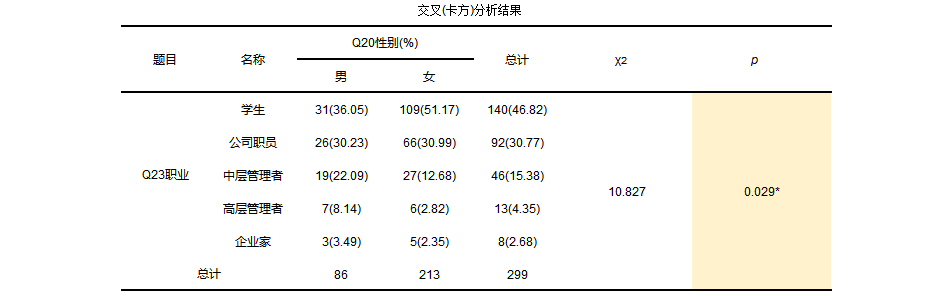From the results, it can be seen that there are more women than men among the respondents, whether male or female students account for 46.82% of the total, and entrepreneurs account for the least. The chi-square value of the model is 10.827, and the p value is about 0.029 less than 0.05 , so it shows that the occupations of respondents of different genders are different.

2. Chi-square analysis of multiple choice questions

Chi-square analysis of multiple-choice questions is theoretically also to study the relationship between two categorical data. The difference is that the independent variable here is single-choice question data, and the dependent variable is multiple-choice question data. You can use SPSSAU [single choice - multiple choice] for analysis. For example, if you want to study "cross-analysis of different incomes and aspects that pay more attention to online courses", the operation is as follows: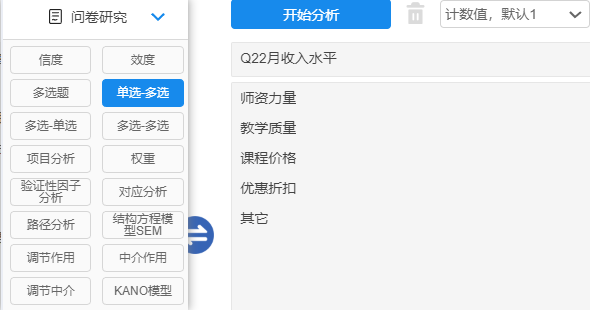The result is as follows: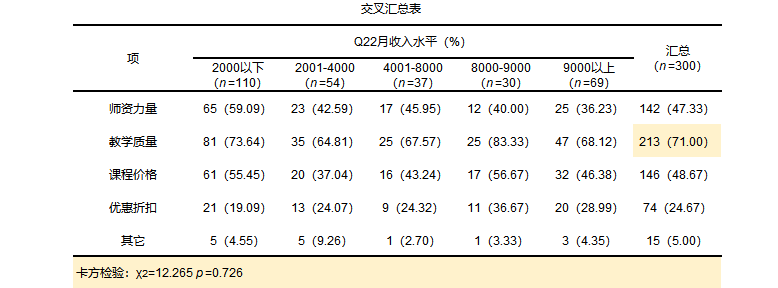From the results, no matter what income level, most people care more about the teaching quality of online courses. A total of 213 people choose this option. Therefore, if you want to manage online courses better, you need to improve the teaching quality, which may be more effective. Finally, it is found that The chi-square value of the square test is 12.265, and the p-value is 0.726 greater than 0.05, so there is no difference in the points of attention to online courses at different income levels. Next, study the influence relationship.

## 3. Analysis of influence relationship

Logit regression analysis is also an analysis of the influence of independent variables on the dependent variable, but the dependent variable of logit regression needs to be a definite variable. General logit regression analysis includes binary logit regression analysis, multi-category logit regression analysis, ordered logit regression analysis, three The difference is as follows: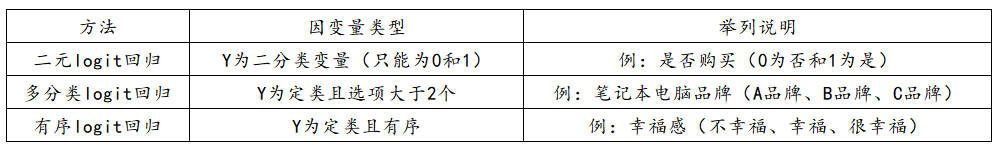1. Binary logit regression analysis

The characteristic of this type of problem is that the dependent variable (Y) is definite data, and only two numbers are used to represent it, which are stipulated as 1 and 0, and can only be 1 or 0. For example, 1 means willing and 0 means unwilling; 1 means 0 means no; 1 means yes; 0 means no; 1 means like and 0 means dislike.
If you want to study the influence of some factors (X) on the dependent variable (Y), and the dependent variable (Y) has only two values ​​(and can only be 0 and 1), then you should use binary Logistic regression analyze. Analysis examples can refer to:

2. Multi-class logit regression

Multi-category logit regression analysis is used to study the relationship between X and Y, where X can also be classified data (if X is classified data, dummy (dummy) variable settings need to be made), and Y is multi-classified data. For multi-class logit regression analysis, it can be divided into three steps.
First: Explain the basic background of the model; for example, the model studies the impact of X on Y, which are X, and what is the specific situation of Y, etc. Second
: Describe the process of model construction and comparison, including analyzing the p-value to detect whether the model construction is meaningful, and the repeated selection process during model construction, using AIC and BIC criteria to compare, and selecting the optimal model, etc.;
: To analyze the specific situation of the model, first analyze the p value, if the value is less than 0.05, it means that X has an influence on Y, and then it is enough to study the influence relationship in detail, such as whether it is a positive influence or a negative influence relationship, etc.; except In addition, you can also write the regression model construction formula, as well as the prediction accuracy of the model, etc.

3. Ordinal logit regression

Ordered Logit regression analysis is used to study the influence relationship between X and Y. If X is classified data, it is generally necessary to set dummy (dummy) variables , and Y is ordered classified data. In orderly Logit regression analysis, first perform the model parallelism test. If the p value is greater than 0.05, it means that the parallelism test is satisfied. If the p value is less than 0.05, it means that the parallelism test is not satisfied. At this time, SPSSAU recommends using multi-category Logit regression analysis; After satisfying the parallelism test, it is enough to study the influence relationship in detail, such as whether it is a positive influence or a negative influence relationship, etc.; in addition, you can also write the model construction formula for ordered Logit regression analysis, as well as the prediction of the model accuracy rate etc.

### Guess you like

Origin blog.csdn.net/m0_37228052/article/details/130385536
Recommended
Ranking
Daily